Smartick is an advanced online program that teaches kids math and coding in only 15 min. a day

Dec10

# Logic Exercises: Graphs

In today’s entry, we are going to explain a type of logic exercise that can sometimes get confusing for students.

These are logic exercises based on images or graphs and next, we will solve one of the logic exercises that can appear during the Smartick logic sessions.

Problem: “The image shows a figure with eight dots joined by segments. At each point, you can write one of the numbers 1, 2, 3, or 4 so that the numbers at each end of the segment are different. Three of the numbers are already shown in the figure. How many times will the number 4 be written when the figure is complete?”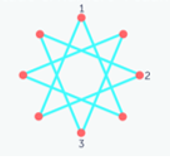If we fail to solve the exercise on the first try, Smartick gives us a clue:

CLUE: “For example, there is a point that can only have a 3 or a 4 because it is joined with a 1 and a 2. Continue this way to complete the figure.”

Following the clue’s indication, we are going to complete the figure. The clue refers to this point: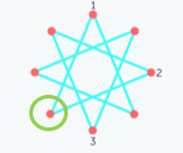We can only put 3 or 4 here because the other ends are a 1 and a 2.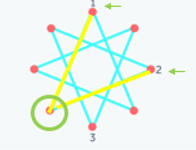Since we want to put as many 4’s as possible, we write a 4 and move to another point where two segments meet with ends already defined by two numbers. Can we put another 4 there?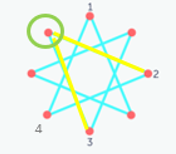The ends are already defined as 3 and 2, so we can put another 4 and continue to the next point. There are no more points with two defined ends, so we take one with only one defined end. Can we put another 4 here?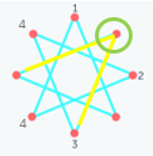Seeing that the fixed end has a 3, we can put a 4 here perfectly and move on to the next point. Can we put 4 again?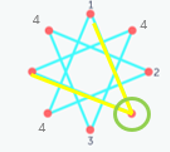This time one of the ends has a 1, so we can put another 4 at this point. We only have one point left, let’s see what number we will be able to put. Will it be a 4?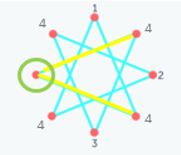Clearly, we cannot put a 4 in this case since the numbers on the two opposite ends are 4.

So, counting how many 4’s we have put, we can see that the answer to the exercise is that the maximum number of times we can put the number 4, is four.

I hope that this post has helped you. If you would like to practice more types of logic exercises like this and many others, head to Smartick and try our method for free.